Subject:
Numbers and Operations
Material Type:
Lesson Plan
Level:
Middle School
7
Provider:
Pearson
Tags:
• Number Line
• Subtraction
Language:
English
Media Formats:
Text/HTML

# Understanding Fractions & Decimals## Overview

Students use number lines to solve addition and subtraction problems involving positive and negative fractions and decimals. They then verify that the same rules they found for integers apply to fractions and decimals as well. Finally, they solve some real-world problems.

# Key Concepts

The first four lessons of this unit focused on adding and subtracting integers. Using only integers made it easier for students to create models and visualize the addition and subtraction process. In this lesson, those concepts are extended to positive and negative fractions and decimals. Students will see that the number line model and rules work for these numbers as well.

Note that rational number will be formally defined in Lesson 15.

# Goals and Learning Objectives

• Extend models and rules for adding and subtracting integers to positive and negative fractions and decimals.
• Solve real-world problems involving addition and subtraction of positive and negative fractions and decimals.

# Lesson Guide

Give partners a few minutes to look at the expression in the Opening and to write word problems it might represent. Ask a few pairs to share their problems.

Some possible problems:

• The low temperature on Saturday was 1.3°F. The low temperature on Sunday was −2.7°F. What was the difference between the low temperatures on Saturday and Sunday?
• Lucy and Jack measured the temperatures of their freezers. Lucy’s freezer is 1.3°F and Jack’s is −2.7°F. Whose freezer is colder? How much colder is it than the other freezer?

# Temperature

Work with your partner to write a word problem about temperature that can be represented by the following expression. You do not need to solve your word problem.

1.3 − (−2.7)

• Share your problem with the class.## Hint:

To write a word problem for the expression, think about the different ways of looking at subtraction.

• You can think of subtraction as “taking away from” or decreasing a starting amount.
• You can think of subtraction as finding the distance between two numbers.

# Lesson Guide

Discuss the Math Mission. Students will use number lines and subtract positive and negative fractions and decimals.

## Opening

Use number lines to add and subtract positive and negative fractions and decimals.

# Lesson Guide

Have students work in pairs.

# Mathematics

You may need to remind students that subtracting a positive number means moving in the negative direction, and subtracting a negative number means moving in a positive direction. Suggest that students check their solutions to see if they are reasonable.

SWD: Decide how you will partner students together for this task. Partnering students by skill level will allow for more efficient provision of teacher support, while partnering students heterogeneously will promote cooperative teaching and learning opportunities for students with varying mathematical skills. Monitor partnerships to ensure all students’ progress. It is often best practice to pair students with disabilities with typically developing peers.

# Interventions

Student draws incorrect models with the Number Line Tool interactive.

• Where should the first arrow start? In which direction should it point?
• Where should the second arrow start? In which direction should it point?
• How long should your second arrow be? Did you check to make sure it has the correct length?

• 2.4 − 4.2 = −1.8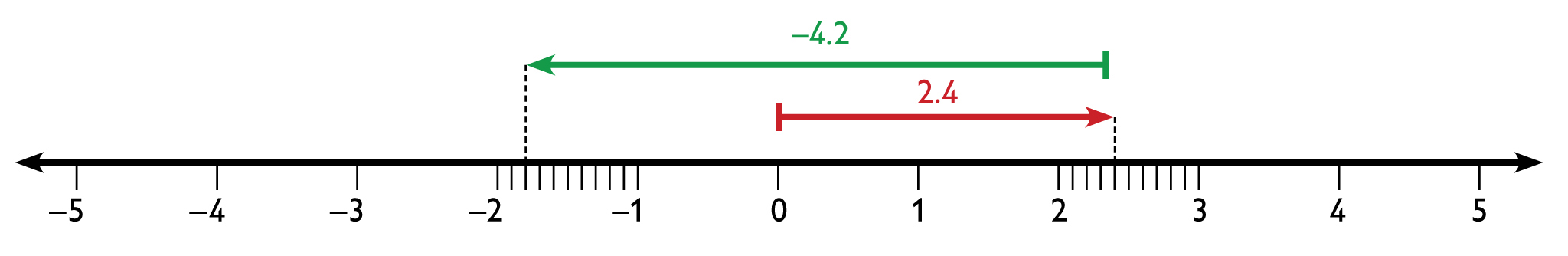• 1.3 − (−2.7) = 4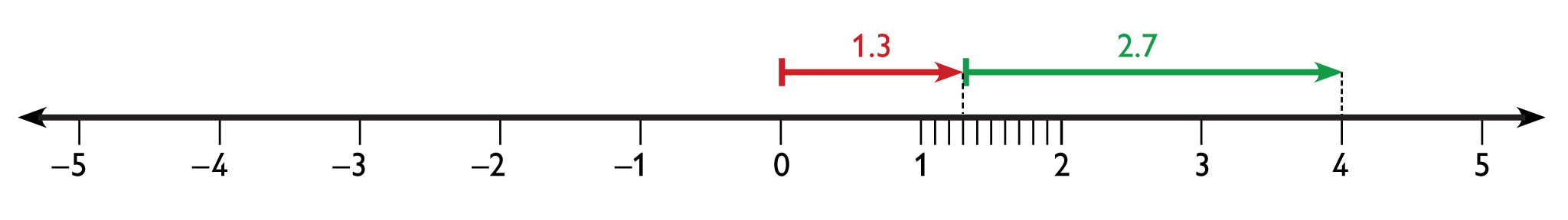• −3.9 + 7.4 = 3.5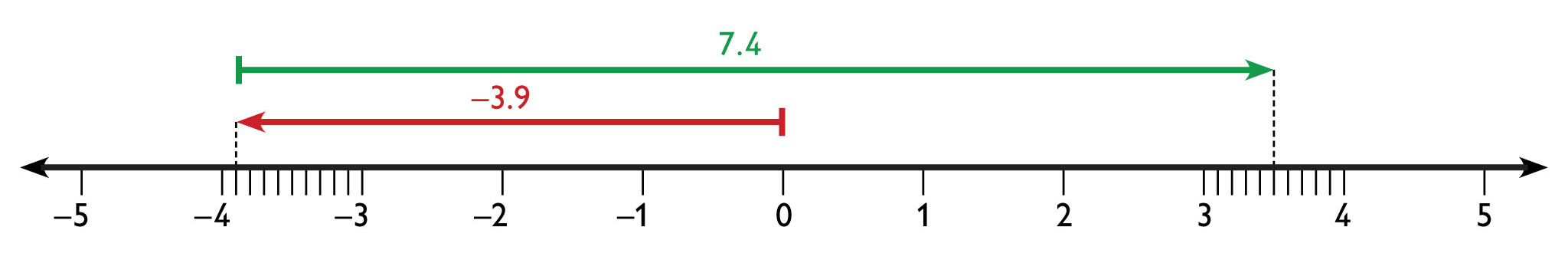## Work Time

Use the Number Line Tool interactive to find each sum or difference. Write the answer as an equation.

• 2.4 − 4.2
• 1.3 − (−2.7)
• −3.9 + 7.4

INTERACTIVE: Number Line Tool

# Lesson Guide

Have students continue to work in pairs.

# Interventions

Student draws incorrect models with the number line.

• Where should the first arrow start? In which direction should it point?
• Where should the second arrow start? In which direction should it point?
• How long should your second arrow be? Did you check to make sure it has the correct length?

• $\frac{3}{4}+\left(-2\frac{1}{2}\right)=-1\frac{3}{4}$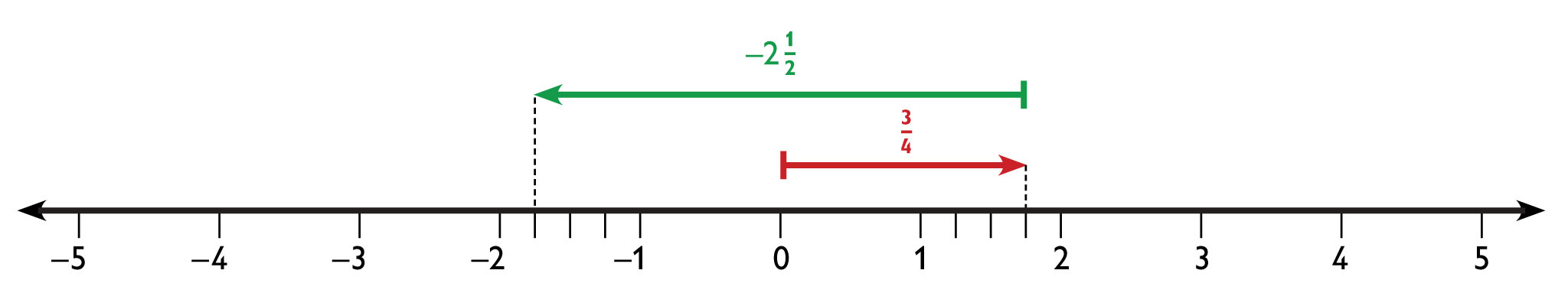• $3\frac{1}{3}-5\frac{2}{3}=-2\frac{1}{3}$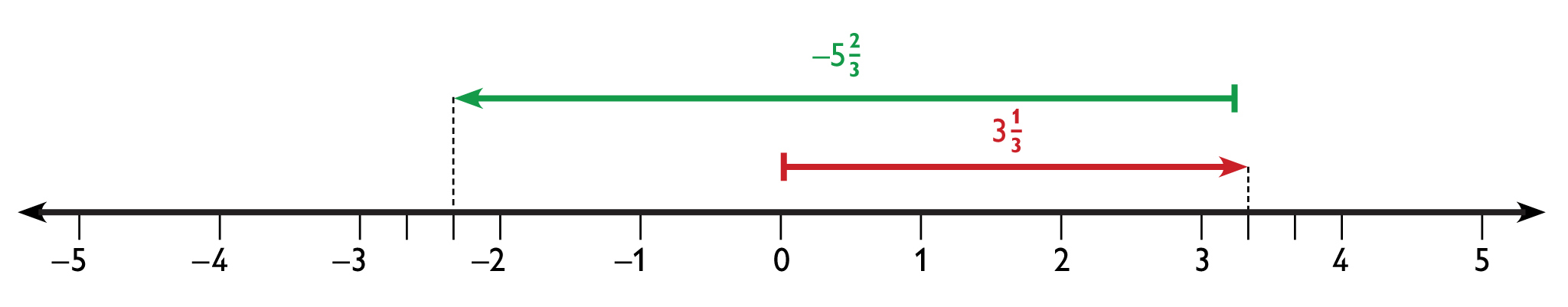• $-4\frac{1}{5}-\left(-3\frac{2}{5}\right)=-\frac{4}{5}$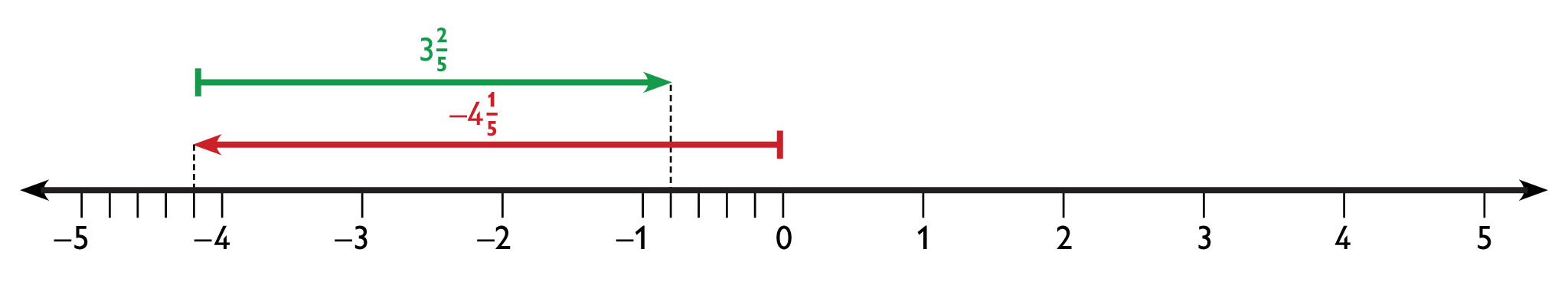## Work Time

Use the Number Line Tool to find each sum or difference. Write the answer as an equation.

• $\frac{3}{4}+\left(-2\frac{1}{2}\right)$
• $3\frac{1}{3}-5\frac{2}{3}$
• $-4\frac{1}{5}-\left(-3\frac{2}{5}\right)$

INTERACTIVE: Number Line Tool

## Hint:

What is the method for adding numbers with different signs?

• Apply this method to the addition problem with different signs from this task or from the previous task.
• Do you get the same answer that you got using the number line?

# Lesson Guide

Have students continue to work in pairs. Encourage students to use a horizontal number line for the checking account problem in order to visualize the problem.

ELL: Be sure students understand the terms balance and penalty in the problem.

Suggest that students check their solutions to see if they are reasonable.

# Mathematical Practices

Mathematical Practice 1: Make sense of problems and persevere in solving them.

Students must analyze the problem to determine what they are given, what they need to find, and how these things are related. They must plan a solution, and after they have solved the problem, look back to see if their answer makes sense.

# Interventions

Student gets an unreasonable answer for a word problem.

• $32.45 −$52.75 − $20 = −$40.30

## Work Time

Kevin writes a check for $52.75, but he has only$32.45 in his checking account.

Because Kevin’s balance is now less than $0, his bank charges him a penalty fee of$20.

• What is the balance in Kevin’s account now?

## Hint:

Start with $32.45. • Do you need to add or subtract$52.75 to represent the check that Kevin wrote?
• Do you need to add or subtract the penalty fee?

# Lesson Guide

Encourage students to use a vertical number line for the elevation problem in order to visualize the problem.

ELL: Be sure students understand the term elevation in the problem.

Suggest that students check their solutions to see if they are reasonable.

# Mathematical Practices

Mathematical Practice 4: Model with mathematics.

Solving these problems requires modeling real-life situations with mathematical expressions and then simplifying the expressions to find the solutions.

# Interventions

Student gets an unreasonable answer for a word problem.

• How can you use estimation to check that your answer is reasonable?

• |$-17\frac{1}{2}$ feet $-19\frac{2}{9}$ feet | = $37\frac{1}{6}$ feet

## Work Time

Sophie and Jack are hiking on the same trail.

Sophie is at an elevation of $-17\frac{1}{2}$ ft.

Jack is at an elevation of $19\frac{2}{3}$ ft.

• How much greater is Jack’s elevation than Sophie’s?## Hint:

Try sketching a number line and showing the two elevations. How can you find the difference between the elevations?

# Lesson Guide

Look for students who demonstrate the subtraction rule for integers, fractions, and decimals. Have these students present during Ways of Thinking. Student presentations should make clear connections between quantitative reasoning (examples) with abstract reasoning (generalization to the subtraction rule).

Have students who solved the Challenge Problem present and explain their solutions during Ways of Thinking as well.

# Mathematical Practices

Mathematical Practice 2: Reason abstractly and quantitatively.

Students must apply the subtraction rule to numerical problems and make connections between them while preparing presentations.

ELL: In working with ELLs during Ways of Thinking:

• Speak clearly and monitor your speech for slang and idiomatic expressions
• Use intonation, volume, and pauses to help make the meaning clear
• Rephrase instead of repeating; paraphrase important concepts and directions

# Challenge Problem

• x = 4.5 and x = −1.1
• x = $\frac{5}{8}$ and x = $\frac{1}{8}$

# Prepare a Presentation

Explain how the methods you used in previous lessons to add and subtract integers also work to add and subtract rational numbers (fractions and decimals). Support your thinking with examples of your work.

# Challenge Problem

Each equation has two solutions. Find both of them.

• |x − 1.7| = 2.8
• $|x-\frac{3}{8}|=\frac{1}{4}$

# Mathematics

Be sure all students understand that the rules for subtracting integers from earlier lessons work for all rational numbers.

If Challenge Problem presenters don’t mention it, remind students that the absolute value of the difference between two numbers is the distance between them. So, for example, the solutions of |x − 1.7| = 2.8 are two points that are 2.8 units away from 1.7. These numbers are 1.7 + 2.8 and 1.7 − 2.8.

# Ways of Thinking: Make Connections

## Hint:

As other students present, ask questions such as:

• Are you sure you moved the correct number of units for the second number?
• Are you sure you moved in the correct direction for the second number?
• How did you decide which operations you needed to perform in order to solve the problem?
• Shouldn’t the answer to that problem be negative?
• How does your explanation compare with that of other students?

# A Possible Summary

You can add and subtract positive and negative fractions and decimals by using a number line in the same way you do for integers. You can also add and subtract using the same rules you used for integers. So, for example, you can subtract a positive or negative fraction or decimal by adding its opposite.

SWD: Scaffold the task of writing a summary into discrete, sequential tasks:

• Identify the big mathematical ideas in the lesson.
• Use a prewriting strategy to organize your thoughts.
• Write a clear, brief explanation of the big ideas in a logical order.
• Refer to the hint questions as a checklist of the information that you need to include in your summary.

Create a digital or paper resource of these tasks for students to keep in front of them as they write their summaries. Some students may get confused navigating between these tasks, the hints, and their written work.

# Summary of the Math: Add and Subtract Positive and Negative Numbers

## Hint:

• Do you explain how you can use the methods for adding and subtracting positive and negative integers to add and subtract positive and negative fractions and decimals?